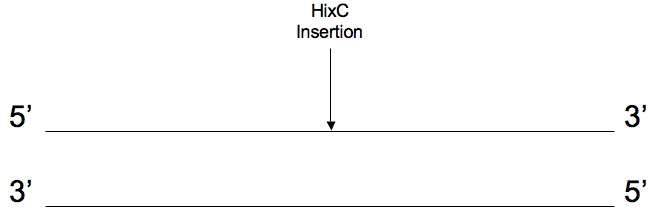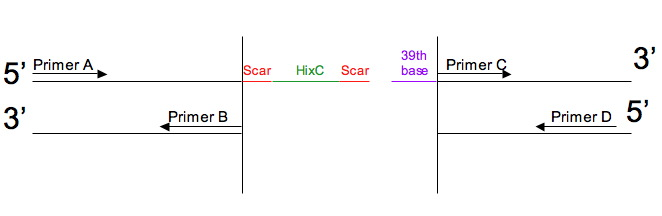# Gene Splitting

## Purpose

This page is designed to tell you what 4 primers will be used to split a gene such that hixC can be inserted within the gene's coding sequence. You can see an example of why you might want to split a gene with hixC, and how this splitting process works for green fluorescent protein (GFP).

Given the DNA sequence from a certain gene (e.g.,GFP), pick the point where you want to split the gene and insert a hixC. A hixC is a 26 base pair long sequence which allows DNA flipping to occur. Because we are using BioBricks, we have a scar of 6 base pairs at each end of the hixC. Therefore, the outcome will be scar + hixC+ scar= 6+26+6 = 38 base pairs in length. This 38 base pair insertion is not a multiple of 3 and therefore disrupts the reading frame after the insertion. Choosing the 39th base will give one of two amino acids, Glu (glutamate) or Asp (aspartate). In addition, picking one of the four bases can slightly modify the melting point in the PCR reaction to make the two halves of the gene. The user can choose which amino acid he or she prefers. A tutorial at the iGEM 2007 wiki can be accessed here.

Before the gene is split it looks like this:The output is a list of the 4 primers used to make the two halves of the gene with PCR (Polymerase Chain Reaction). The software generates the 4 primers shown below:

• Primer A is the begining of the first half of the gene.
• Primer B is the end of the complement of the first half of the gene.
• Primer C is the begining of the second half of the gene.
• Primer D is the end of the complement of the second half of the gene.

After the gene is split it looks like this:Primers will be optimized so that the melting temperature for Primer A and Primer B are as close as possible. Also, the melting temperature for Primer C and Primer D should be as close as possible. Melting temperature (Tm) is calculated by the following algorithm:

If the length of the strand is 20 or less:

Return 4*(# Gs + # Cs) + 2*(# As + # Ts).

Otherwise:

Compute sigma_dH and sigma_dS based on enthalpy and entropy values for base-pairs. Return (-1000 * sigma_dH / (-10.8 - sigma_dS + 1.987 * -23.5) - 273.15 + 16.6 * -1.3).

## Directions :

1. Enter the gene's DNA sequence.
2. Enter the number of the last amino acid of the first half of the protein.
3. Pick the 39th base (to be added at the 5' end of primer C).
4. Click on the submit button.

## References

Acker, Karen. Splitting Genes to Make Multiple Pancakes, independent study final paper (2007).